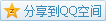# MIT OCW

## 18.02 Multivariable Calculus

### Lagrange Multiplier

We've known the method to maximize or minimize a multivariable function, but what happens if there are some constraint? The critical point usually does not fulfill the constraint, so we have to maximize or minimize in another way.

#### Introduction

e.g. Find the closest point to origin on $xy=3$.
Idea We are going to minimize $f(x,y)=x^2+y^2$ subject to $xy=3$. These are the level surfaces of $f(x,y)$ and $g(x,y)=xy=3$.When the level surface $f(x,y)=c$ becomes smaller and smaller, until it has no intersection with the $xy=3$, then we have almost achieved the goal. We can find that at the maximum or minimum $f_0$, the level surface $f(x,y)=f_0$ is tangent to the level surface $g(x,y)=3$.It means that the gradient at $f(x,y)$ is parallel to $g(x,y)$, namely, $\nabla f=\lambda\nabla g$, where $\lambda$ is an unknown. So, we have such system of equations \left\{\begin{aligned}2x=\lambda y\\2y=\lambda x\\xy=3\end{aligned}\right., and we get the point is $(\sqrt3,\sqrt3)$ or $(-\sqrt3,-\sqrt3)$.

#### Lagrange Multiplier

Now we conclude the method, given a function $f(x,y)$ and a constraint $g(x,y)=c$, then to maximize or minimize the function, we need to solve the system of equations \left\{\begin{aligned}\frac{\partial f}{\partial x}&=\lambda\frac{\partial g}{\partial x}\\\frac{\partial f}{\partial y}&=\lambda\frac{\partial g}{\partial y}\\g(x,y)&=c\end{aligned}\right..

#### Geometry

Why it is correct? If there is no constraint $g(x,y)=c$, we just solve $f_x=f_y=0$, and it means when we move on a horizontal surface near the point, the function doesn't change.
Now we have a constraint, similarly, we just find a point where we move along the constraint surface that the function doesn't change too. So $\nabla_{\hat\mathbf{u}}f=0$, where $\hat\mathbf{u}$ is any direction on the constraint surface, in other words, $\nabla f\cdot\hat\mathbf{u}=0$. Since the gradient $\nabla g$ is also normal to the constraint surface, we have $\nabla f\parallel\nabla g$.

#### Application

e.g. Find a best solution to minimizing the surface area of a pyramid with a given triangular base $a_1,a_2,a_3$ and a given height $h$.
Solution We can plot the pyramid in $xy$ plane.And it looks like the following in 3D space.To determine the position of the vertex $D$ more easily, we project the vertex on the $xy$ plane.And take the distance from the projection to three sides $a_1,a_2,a_3$ as $d_1,d_2,d_3$, and we can express the surface area $S$ and the base area $A$: \begin{aligned}S=\frac12a_1\sqrt{d_1^2+h^2}+\frac12a_2\sqrt{d_2^2+h^2}+\frac12a_3\sqrt{d_3^2+h^2}\\A=\frac12a_1d_1+\frac12a_2d_2+\frac12a_3d_3\end{aligned}.
And apply the Lagrange Multiplier \left\{\begin{aligned}\frac{\partial S}{\partial d_1}=\lambda\frac{\partial A}{\partial d_1}\\\frac{\partial S}{\partial d_2}=\lambda\frac{\partial A}{\partial d_2}\\\frac{\partial S}{\partial d_3}=\lambda\frac{\partial A}{\partial d_3}\end{aligned}\right., and found that $d_1=d_2=d_3$, so the vertex is just above the incenter of the triangular base.